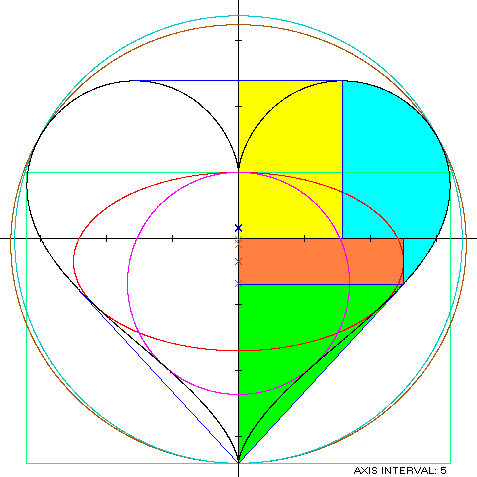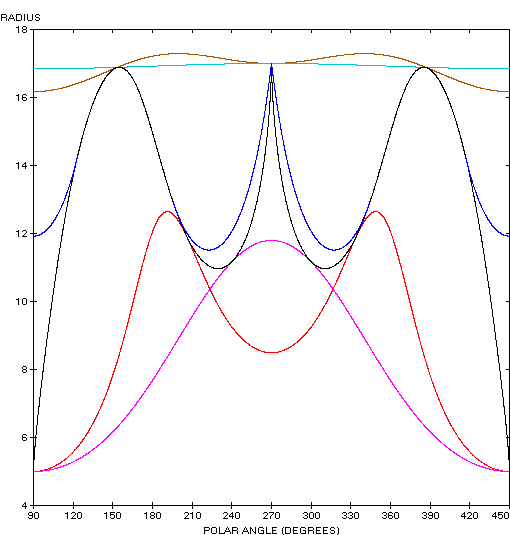Heart Curve
HEART CURVE

Balmoral Software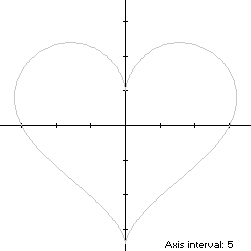The fifth heart curve is an algebraic eighth-degree y-symmetric closed curve S defined by the parametric equations
x(t) = 16sin3(t)

y(t) = 13cos(t) - 5cos(2t) - 2cos(3t) - cos(4t)

for t ∈ T = [0,2π). The curve follows a clockwise path as t increases, starting from the cusp (0,5) at t = 0, reaching its maximum-abscissa point (16,4) at t = π/2, its minimum-ordinate point (0,-17) at t = π, and then following a symmetric path back to the cusp. Since maximum-ordinate points (±7.838207,11.923252) occur at
t1 = 0.908063
and at -t1 in the two bulges of the heart, S is non-convex by the multiple local extrema test, and the width x height of its bounding rectangle is 32 x 28.923252.

### Metrics

We have
x'(t) = 48sin2(t)cos(t)

y'(t) = -13sin(t) + 10sin(2t) + 6sin(3t) + 4sin(4t),

so by (L1), the perimeter of the heart curve is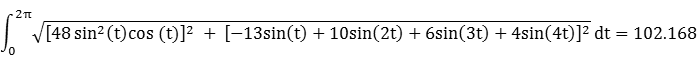and its area is A = 180π. By (C1), its centroid ordinate is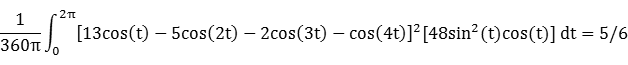The bounding rectangle for inconics is delimited by the cusp at (0,5).

### Convex Hull

The top part of the convex hull of the heart curve is created by connecting the two maximum-ordinate points with a line segment of length 2x(t1) = 15.676414. We have
x''(t) = -12sin(t) + 36sin(3t)

y''(t) = -13cos(t) + 20cos(2t) + 18cos(3t) + 16cos(4t),

so by (X1), the curvature of S changes sign at (0,-17) since
48sin2(t)cos(t)[-13cos(t) + 20cos(2t) + 18cos(3t) + 16cos(4t)] = [-12sin(t) + 36sin(3t)][-13sin(t) + 10sin(2t) + 6sin(3t) + 4sin(4t)]
is satisfied for t = π. Equation (X2) is evaluated with respect to the point (0,-17) as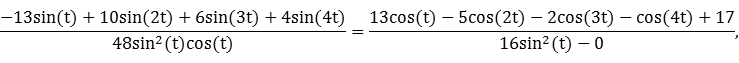which has a solution in the right half-plane at
t2 = 1.973512,
so convex hull line segments extend between the points (0,-17) and (±x(t2),y(t2)) = (±12.459008,-3.460803). These line segments are shown in dark blue at the bottom of the left diagram below, and each has length 18.399368. By (L1), the perimeter of the convex hull is: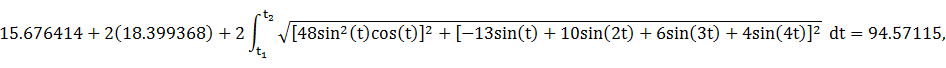which is about 7% shorter than that of the heart curve.

The right half of the convex hull can be partitioned into four areas, shown in color in the left diagram below:

A rectangle P1 (yellow) with corners at the origin and at the maximum-ordinate point (x(t1),y(t1)).

A right trapezoid containing all the Quadrant IV space between the lower-right convex hull line segment and the vertical line at x(t2). For convenience, the trapezoid is separated into a rectangle P2 (orange) with corners at the origin and at (x(t2),y(t2)), and a triangle P3 (green) containing the remainder of the trapezoid.

A comma-shaped region P4 (light blue) delimited by the curve S between t1 and t2 and the boundaries of P1 and P2.

The centroid ordinate of each rectangle and triangle is the average of its vertex ordinates. By (A1), the area of the comma-shaped region P4 is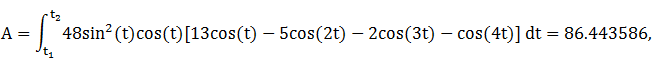and by (C1), its centroid ordinate is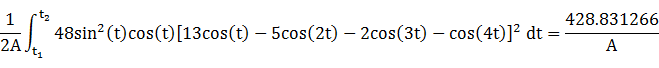The half-hearted convex hull component metrics can be summarized as follows:
RegionAreaCentroid ordinateProduct
P1x(t1)y(t1) = 93.456921y(t1)/2x(t1)y2(t1)/2 = 557.155231
P2∣x(t2)y(t2)∣ = 43.118171y(t2)/2-x(t2)y2(t2)/2 = -74.611741
P3x(t2)[y(t2) + 17]/2 = 84.342486[2y(t2) - 17]/3x(t2)[y(t2) + 17][2y(t2) - 17]/6 = -672.535896
P4A = 86.443586428.831266/A428.831266
Total307.361164238.838860
The area of the entire convex hull then is 614.722328, about 9% larger than the area of the heart curve. The centroid ordinate of the convex hull is the weighted average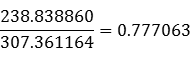### Incircle

Using z = 5 in Lemma C, we have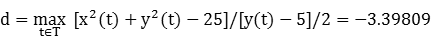and R = |d - z| = 8.39809. For verification, we have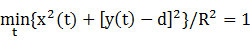### Inellipse

Using z = 5 in Lemma E,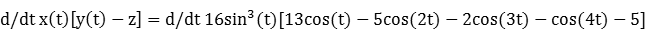has a zero at t* = 2.071360. The corresponding coordinates are
x* = 10.8039

y* = -5.11923

We then have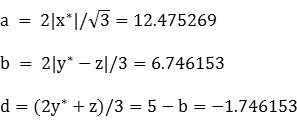For verification,### Circumellipse

Using z = -17 in Lemma E,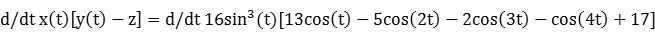has a zero at t* = 1.355197. The corresponding coordinates are
x* = 14.9143

y* = 7.8781

We then haveFor verification,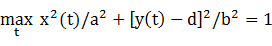### Circumcircle

Using z = -17 in Lemma C,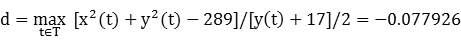and R = |d - z| = 16.922074. For verification, we have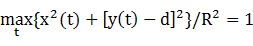The optimal circumcircle is slightly smaller than an origin-centered circle with radius = 17 exactly.

### Summary Table

 Perimeter Area Centroid Figure Parameters Incircle R = 8.39809 52.766756 221.569982 (0,-3.39809) Inellipse a = 12.475269b = 6.746153 61.734653 264.396668 (0,-1.746153) Heart curve Width: 32Height: 28.923252 102.168 565.486678 (0,0.833333) Convex hull 94.57115 614.722328 (0,0.777063) Circumellipse a = 17.221550b = 16.585373 106.216983 897.320010 (0,-0.414627) Circumcircle R = 16.922074 106.324527 899.615755 (0,-0.077926)